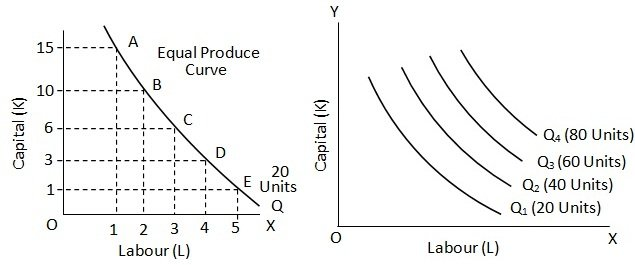Isoquants

# Isoquants

It is well – known that factors of production are to some extent substitutes for each other. Whatever can be produced with more of labour and less of capital, can also be produced with less of labour and more of capital. Thus, to an extent labour can be used in place of capital and vice versa to obtain the same given level of output. An equal product curve shows all those input (factor) combinations which give the same level of output. Let us assume that there are only two factors, viz. labour (L) and capital (K) and the various combinations of these which give the same level of output.

Factor Combinations Yielding Equal Product

 Factor Combination Labour Capital A B C D E 1 2 3 4 5 15 10 6 3 1

It is clear that combination A used more of capital and less of labour and combination E more of labour and less of capital to produce the same level of output. In between these two are the combinations B, C and D using varying amounts of capital and labour and giving the same output. If we plot all these combinations and join the various points showing these combinations, we get the equal product curve.

The equal product curve is also called Isoquants or Iso – product curve since it represents combinations of inputs which are capable of yielding equal quantity of output.An increase in the quantity of both the inputs, viz, labour and capital, would yield a larger quantity of output and hence the isoquant would move upward. Thus, we can draw a number of Isoquants, one above the other, showing each higher level of output that could be obtained by various combinations of labour and capital. As shows Q1, Q2, Q3, etc. are the Isoquants showing successively higher quantities of output.

### Submit Homework

Submit your homework for a free quote